Updating search results...

# 25 Results

View
Selected filters:
• CCSS.Math.Content.6.EE.B.6Rating
4.0 stars

Use the Sound Grapher to create visualizations of sound and learn about the frequency, wavelength, amplitude and velocity of sound waves.

Subject:
Chemistry
Physics
Material Type:
Activity/Lab
Lecture Notes
Provider:
Concord Consortium
Provider Set:
Concord Consortium Collection
Author:
The Concord Consortium
12/11/2011Conditional Remix & Share Permitted
CC BY-NC
Rating
0.0 stars

During this two-day lesson, students work with a partner to create and implement a problem-solving plan based on the mathematical concepts of rates, ratios, and proportionality. Students analyze the relationship between different-sized gummy bears to solve problems involving size and price.Key ConceptsThroughout this unit, students are encouraged to apply the mathematical concepts they have learned over the course of this year to new settings. Helping students develop and refine these problem solving skills:Creating a problem solving plan and implementing their plan systematicallyPersevering through challenging problems to find solutionsRecalling prior knowledge and applying that knowledge to new situationsMaking connections between previous learning and real-world problemsCommunicating their approaches with precision and articulating why their strategies and solutions are reasonableCreating efficacy and confidence in solving challenging problems in a real worldGoals and Learning ObjectivesCreate and implement a problem-solving plan.Organize and interpret data presented in a problem situation.Analyze the relationship between two variables.Use ratios.Write and solve proportions.Create rate tables to organize data and make predictionsUse multiple representations—including tables, graphs, and equations—to organize and communicate data.Articulate strategies, thought processes, and approaches to solving a problem and defend why the solution is reasonable.

Subject:
Ratios and Proportions
Material Type:
Lesson Plan
Provider:
Pearson
09/21/2015Conditional Remix & Share Permitted
CC BY-NC
Rating
0.0 stars

Lesson OverviewStudents apply the multiplication property of equality to solve equations.Key ConceptsIn the previous lesson, students solved equations of the form x + p = q using the addition property of equality. In this lesson, they will solve equations of the form px = q using the multiplication property of equality. They will multiply or divide both sides of an equation by the same number to obtain an equivalent equation.Since multiplication by a is equivalent to division by 1a, students will see that they may also divide both sides of the equation by the same number to get an equivalent equation. Students will also apply this property to solving a particular kind of equation, a proportion.Goals and Learning ObjectivesUse the multiplication property of equality to keep an equation balanced.Use the multiplication property of equality to solve equations of the form px = q for cases in which p, q, and x are all non-negative rational numbers.Use the multiplication property of equality to solve proportions.

Subject:
Algebra
Material Type:
Lesson Plan
Provider:
Pearson
09/21/2015Only Sharing Permitted
CC BY-NC-ND
Rating
4.0 stars

This lesson unit is intended to help you assess how well students are able to: Perform arithmetic operations, including those involving whole-number exponents, recognizing and applying the conventional order of operations; Write and evaluate numerical expressions from diagrammatic representations and be able to identify equivalent expressions; apply the distributive and commutative properties appropriately; and use the method for finding areas of compound rectangles.

Subject:
Geometry
Material Type:
Assessment
Lesson Plan
Provider:
Shell Center for Mathematical Education
Provider Set:
Mathematics Assessment Project (MAP)
04/26/2013Unrestricted Use
CC BY
Rating
0.0 stars

In this task students are asked to write an equation to solve a real-world problem. There are two natural approaches to this task. In the first approach, students have to notice that even though there is one variable, namely the number of firefighters, it is used in two different places. In the other approach, students can find the total cost per firefighter and then write the equation.

Subject:
Mathematics
Material Type:
Activity/Lab
Provider:
Illustrative Mathematics
Provider Set:
Illustrative Mathematics
Author:
Illustrative Mathematics
05/01/2012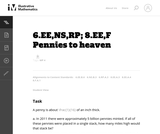Unrestricted Use
CC BY
Rating
0.0 stars

This is a task from the Illustrative Mathematics website that is one part of a complete illustration of the standard to which it is aligned. Each task has at least one solution and some commentary that addresses important asects of the task and its potential use. Here are the first few lines of the commentary for this task: A penny is about $\frac{1}{16}$ of an inch thick. In 2011 there were approximately 5 billion pennies minted. If all of these pennies were placed in a s...

Subject:
Mathematics
Material Type:
Activity/Lab
Provider:
Illustrative Mathematics
Provider Set:
Illustrative Mathematics
Author:
Illustrative Mathematics
03/17/2013Conditional Remix & Share Permitted
CC BY-NC
Rating
0.0 stars

Subject:
Mathematics
Material Type:
Full Course
Provider:
Pearson
10/06/2016Rating
0.0 stars

Getting Started

Type of Unit: Introduction

Prior Knowledge

Students should be able to:

Solve and write numerical equations for whole number addition, subtraction, multiplication, and division problems.
Use parentheses to evaluate numerical expressions.
Identify and use the properties of operations.

Lesson Flow

In this unit, students are introduced to the rituals and routines that build a successful classroom math community and they are introduced to the basic features of the digital course that they will use throughout the year.

An introductory card sort activity matches students with their partner for the week. Then over the course of the week, students learn about the lesson routines: Opening, Work Time, Ways of Thinking, Apply the Learning, Summary of the Math, and Reflection. Students learn how to present their work to the class, the importance of taking responsibility for their own learning, and how to effectively participate in the classroom math community.

Students then work on Gallery problems to further explore the program’s technology resources and tools and learn how to organize their work.

The mathematical work of the unit focuses on numerical expressions, including card sort activities in which students identify equivalent expressions and match an expression card to a word card that describes its meaning. Students use the properties of operations to identify equivalent expressions and to find unknown values in equations.

Subject:
Mathematics
Material Type:
Unit of Study
Provider:
PearsonRating
0.0 stars

Equations and Inequalities

Type of Unit: Concept

Prior Knowledge

Students should be able to:

Add, subtract, multiply, and divide with whole numbers, fractions, and decimals.
Use the symbols <, >, and =.
Evaluate expressions for specific values of their variables.
Identify when two expressions are equivalent.
Simplify expressions using the distributive property and by combining like terms.
Use ratio and rate reasoning to solve real-world problems.
Order rational numbers.
Represent rational numbers on a number line.

Lesson Flow

In the exploratory lesson, students use a balance scale to find a counterfeit coin that weighs less than the genuine coins. Then continuing with a balance scale, students write mathematical equations and inequalities, identify numbers that are, or are not, solutions to an equation or an inequality, and learn how to use the addition and multiplication properties of equality to solve equations. Students then learn how to use equations to solve word problems, including word problems that can be solved by writing a proportion. Finally, students connect inequalities and their graphs to real-world situations.

Subject:
Mathematics
Algebra
Material Type:
Unit of Study
Provider:
PearsonConditional Remix & Share Permitted
CC BY-NC
Rating
0.0 stars

Students discuss as a class the important ways that listeners contribute to mathematical discussions during Ways of Thinking presentations. Students then use the properties of operations to find the value of each fruit used in equations.Key ConceptsStudents use the properties of operations to find the value of each fruit used in different equations. By considering several equations, students can match each of the 10 fruits to the whole numbers 0 through 9. This work helps students see why representing unknown numbers with letters is useful.Goals and Learning ObjectivesContribute as listeners during the Ways of Thinking discussion.Identify the whole numbers that make an equation true.Use the properties of operations, when appropriate, to justify which whole numbers represent unknown values.

Subject:
Algebra
Material Type:
Lesson Plan
Provider:
Pearson
09/21/2015Conditional Remix & Share Permitted
CC BY-NC
Rating
0.0 stars

Lesson OverviewStudents practice solving equations using either the addition or the multiplication property of equality.Key ConceptsStudents will solve equations of the form x + p = q using the addition property of equality.They will solve equations of the form px = q using the multiplication property of equality.They will need to look at the variable and decide what operation must be performed on both sides of the equation in order to isolate the variable on one side of the equation.If a number has been added to the variable, they will subtract that number from both sides of the equation. If a number has been subtracted from the variable, they will add that number to both sides of the equation. If the variable has been multiplied by a number, students will either divide both sides of the equation by that number or multiply by the reciprocal of that number. If the variable has been divided by a number, students will multiply by that number. Students will see how this can be applied to solving a proportion such as xc=ab.Goals and Learning ObjectivesPractice solving equations using either the addition or the multiplication property of equality.Distinguish between equations that can be solved using the addition property of equality from equations that can be solved using the multiplication property of equality.Solve a proportion by solving an equation.

Subject:
Algebra
Material Type:
Lesson Plan
Provider:
Pearson
09/21/2015Conditional Remix & Share Permitted
CC BY-NC
Rating
0.0 stars

During this two-day lesson, students work with a partner to create and implement a problem-solving plan based on the mathematical concepts of rates, ratios, and proportionality. Students analyze the relationship between different-sized gummy bears to solve problems involving size and price.Key ConceptsThroughout this unit, students are encouraged to apply the mathematical concepts they have learned over the course of this year to new settings. Help students develop and refine these problem-solving skills:Creating a problem solving plan and implementing their plan systematicallyPersevering through challenging problems to find solutionsRecalling prior knowledge and applying that knowledge to new situationsMaking connections between previous learning and real-world problemsCommunicating their approaches with precision and articulating why their strategies and solutions are reasonableCreating efficacy and confidence in solving challenging problems in a real worldGoals and Learning ObjectivesCreate and implement a problem-solving plan.Organize and interpret data presented in a problem situation.Analyze the relationship between two variables.Use ratios.Write and solve proportions.Create rate tables to organize data and make predictions.Use multiple representations—including tables, graphs, and equations—to organize and communicate data.Articulate strategies, thought processes, and approaches to solving a problem, and defend why the solution is reasonable.

Subject:
Ratios and Proportions
Material Type:
Lesson Plan
Provider:
Pearson
09/21/2015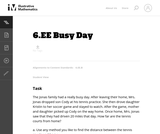Unrestricted Use
CC BY
Rating
3.0 stars

This task provides a good entry point for students into representing quantities in contexts with variables and expressions and building equations that reflect the relationships presented in the context.

Subject:
Mathematics
Material Type:
Activity/Lab
Provider:
Illustrative Mathematics
Provider Set:
Illustrative Mathematics
Author:
Illustrative Mathematics
08/15/2012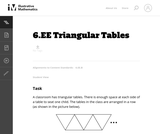Unrestricted Use
CC BY
Rating
0.0 stars

This task provides a good opportunity for group work and class discussions where students generate and compare equivalent expressions. In class discussion, students should be asked to connect the terms of an expression with quantities shown in the diagram.

Subject:
Mathematics
Material Type:
Activity/Lab
Provider:
Illustrative Mathematics
Provider Set:
Illustrative Mathematics
Author:
Illustrative Mathematics
05/01/2012Conditional Remix & Share Permitted
CC BY-NC
Rating
0.0 stars

Lesson OverviewStudents solve problems using equations of the form x + p = q and px = q, as well as problems involving proportions.Key ConceptsStudents will extend what they know about writing expressions to writing equations. An equation is a statement that two expressions are equivalent. Students will write two equivalent expressions that represent the same quantity. One expression will be numerical and the other expression will contain a variable.It is important that when students write the equation, they define the variable precisely. For example, n represents the number of minutes Aiko ran, or x represents the number of boxes on the shelf.Students will then solve the equations and thereby solve the problems.Students will solve proportion problems by solving equations. This makes sense because a proportion such as xa=bc is really just an equation of the form xp = q where p=1a and q=bc.Students will also compare their algebraic solutions to an arithmetic solution for the problem. They will see, for example, that a problem that might be solved arithmetically by subtracting 5 from 78 can also be solved algebraically by solving x + 5 = 78, where 5 is subtracted from both sides—a parallel solution to subtracting 5 from 78.Goals and Learning ObjectivesUse equations of the form x + p = q and xp = q to solve problems.Solve proportion problems using equations.ELL: ELLs may have difficulty verbalizing their reasoning, particularly because word problems are highly language dependent. Accommodate ELLs by providing extra time for them to process the information. Note that this problem is a good opportunity for ELLs to develop their literacy skills since it incorporates reading, writing, listening, and speaking skills. Encourage students to challenge each others' ideas and justify their thinking using academic and specialized mathematical language.

Subject:
Algebra
Material Type:
Lesson Plan
Provider:
Pearson
09/21/2015Conditional Remix & Share Permitted
CC BY-NC
Rating
0.0 stars

Students create and implement a problem-solving plan to solve another problem involving the relationship between the sound of thunder and the distance of the lightning.Key ConceptsThroughout this unit, students are encouraged to apply the mathematical concepts they have learned over the course of this year to new settings. Help students develop and refine these problem-solving skills:Creating a problem-solving plan and implementing their plan systematicallyPersevering through challenging problems to find solutionsRecalling prior knowledge and applying that knowledge to new situationsMaking connections between previous learning and real-world problemsCommunicating their approaches with precision and articulating why their strategies and solutions are reasonableCreating efficacy and confidence in solving challenging problems in a real worldGoals and Learning ObjectivesCreate and implement a problem-solving plan.Organize and interpret data presented in a problem situation.Analyze the relationship between two variables.Create a rate table to organize data and make predictions.Apply the relationship between the variables to write a mathematical formula and use the formula to solve problems.Create a graph to display proportional relationships and use this graph to make predictions.Articulate strategies, thought processes, and approaches to solving a problem and defend why the solution is reasonable.

Subject:
Mathematics
Material Type:
Lesson Plan
Provider:
Pearson
09/21/2015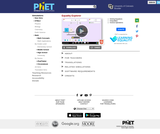Unrestricted Use
CC BY
Rating
0.0 stars

Explore what it means for a mathematical statement to be balanced or unbalanced by interacting with objects on a balance. Discover the rules for keeping it balanced. Collect stars by playing the game!

Subject:
Mathematics
Material Type:
Simulation
Provider:
Provider Set:
PhET Interactive Simulations
Author:
David Wood
Marleene Buttice
Meenakshi Raghavan
03/06/2019
Remix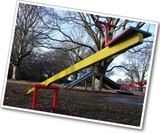Conditional Remix & Share Permitted
CC BY-NC
Rating
0.0 stars

Gallery OverviewAllow students who have a clear understanding of the content thus far in the unit to work on Gallery problems of their choosing. You can then use this time to provide additional help to students who need review of the unit's concepts or to assist students who may have fallen behind on work.Gallery DescriptionsKeep It BalancedStudents will use reasoning to complete some equations to make them true.Equation SortStudents will sort equations into three groups: equations with one solution, equations with many solutions, and equations with no solutionsOn the Number LineStudents will use a number line to identify numbers that make an equation or inequality true.How Many Colors?Students will write and solve an equation to find the number of different colored blocks in a box.Value of sStudents will use a property of equality to solve an equation with large numbers.Marbles in a CupStudents are given information about the weight of a cup with two different amounts of marbles in it. They use this information to find the weight of the cup.When Is It True?Students will use what they know about 0 and 1 to decide when a certain equation is true.

Subject:
Algebra
Material Type:
Lesson Plan
Author:
Amber Delliger
12/12/2017Conditional Remix & Share Permitted
CC BY-NC
Rating
0.0 stars

Lesson OverviewStudents represent real-world situations using inequality statements that include a variable.Key ConceptsInequality statements tell you whether values in a situation are greater than or less than a given number and also tell you whether values in the situation can be equal to that number or not.The symbols < and > tell you that the unknown value(s) in a situation cannot be equal to a given number: the unknown value(s) are strictly greater than or less than the number. The inequality x < y means x must be less than y. The inequality x > y means x must be greater than y.The symbols ≤  and ≥ tell you that the unknown value(s) in a situation can also be equal to a given number: the unknown value(s) are less than or equal to, or greater than or equal to, the number. The inequality x ≤ y means x is less than or equal to y. The inequality x ≥ y means x is greater than or equal to y.Goals and Learning ObjectivesUnderstand the inequality symbols <, >, ≤, and ≥.Write inequality statements for real-world situations.ELL: When writing the summary, provide ELLs access to a dictionary and give them time to discuss their summary with a partner before writing, to help them organize their thoughts. Allow ELLs who share the same primary language to discuss in their native language if they wish.

Subject:
Numbers and Operations
Material Type:
Lesson Plan
Provider:
Pearson
09/21/2015Conditional Remix & Share Permitted
CC BY-NC
Rating
0.0 stars

Lesson OverviewStudents represent inequalities on a number line, find at least one value that makes the inequality true, and write the inequality using words.SWD:When calling on students, be sure to call on ELLs and to encourage them to actively participate. Understand that their pace might be slower or they might be shy or more reluctant to volunteer due to their weaker command of the language.SWD:Thinking aloud is one strategy for making learning visible. When teachers think aloud, they are externalizing their internal thought processes. Doing so may provide students with insights into mathematical thinking and ways of tackling problems. It also helps to model accurate mathematical language.Key ConceptsInequalities, like equations, have solutions. An arrow on the number line—pointing to the right for greater values and to the left for lesser values—can be used to show that there are infinitely many solutions to an inequality.The solutions to x < a are represented on the number line by an arrow pointing to the left from an open circle at a.Example: x < 2The solutions to x > a are represented on the number line with an arrow pointing to the right from an open circle at a.Example: x > 2The solutions to x ≤ a are represented on the number line with an arrow pointing to the left from a closed circle at a.Example: x ≤ 2The solutions to x ≥ a are represented on the number line with an arrow pointing to the right from a closed circle at a.Example: x ≥ 2Goals and Learning ObjectivesRepresent an inequality on a number line and using words.Understand that inequalities have infinitely many solutions.

Subject:
Numbers and Operations
Material Type:
Lesson Plan
Provider:
Pearson